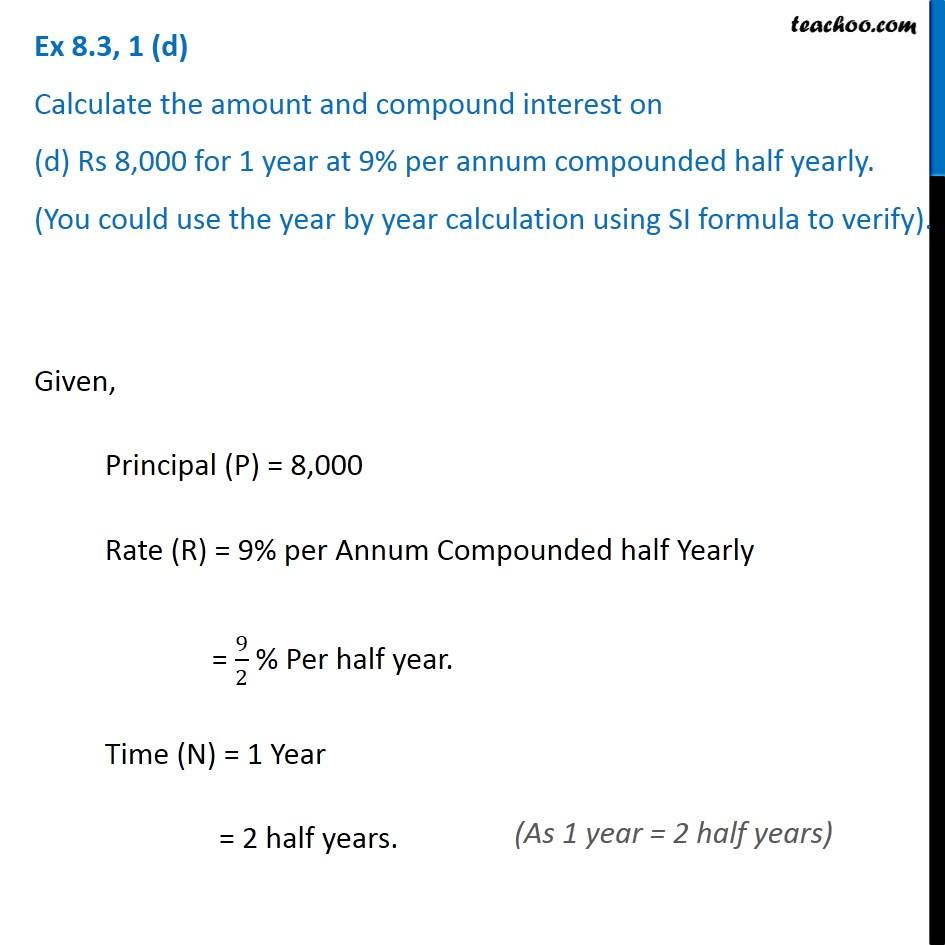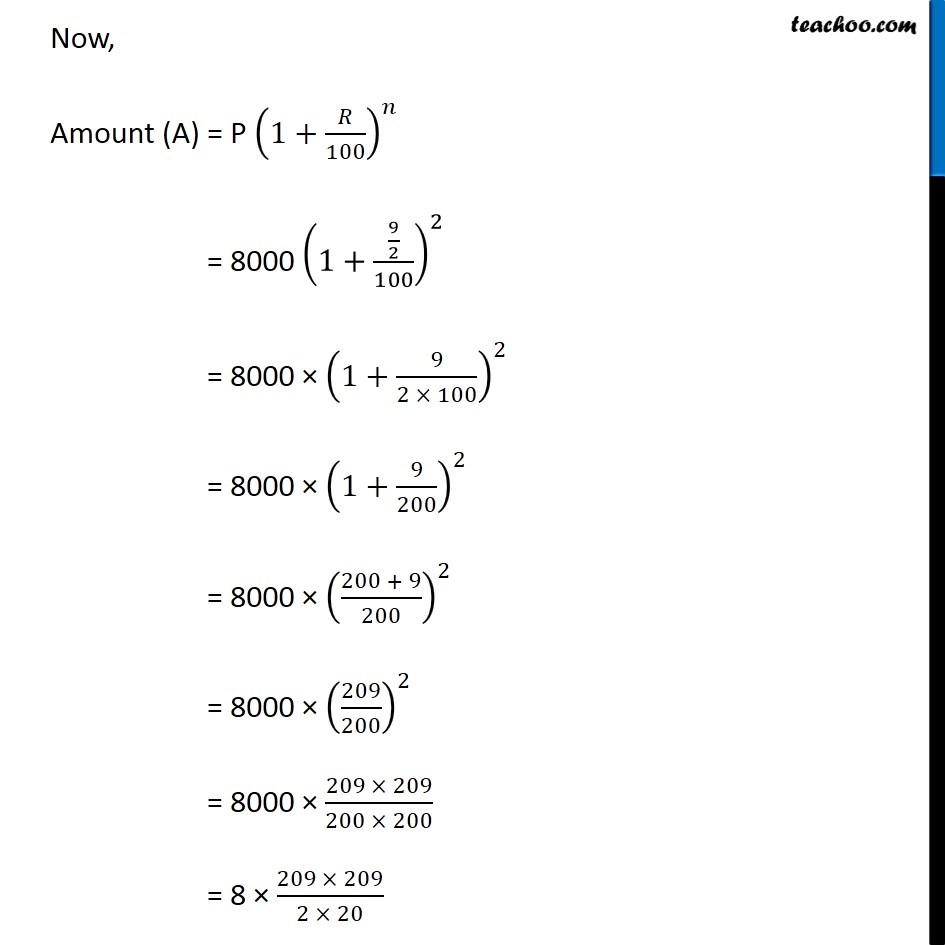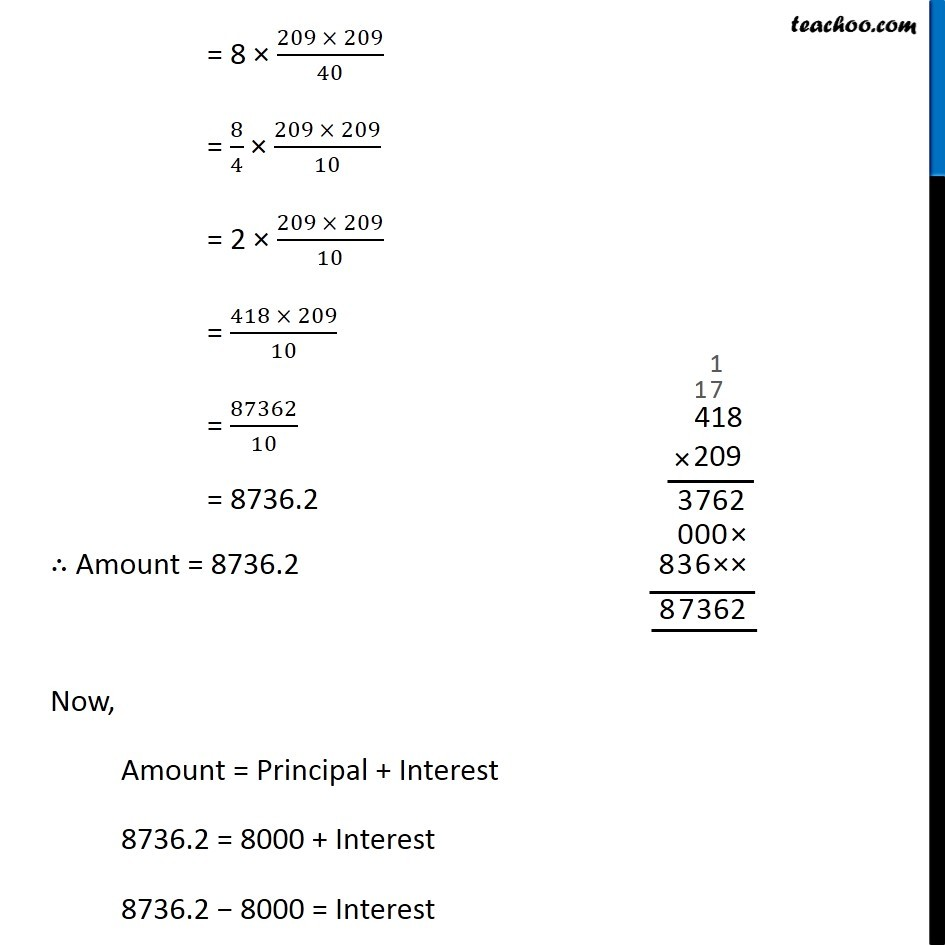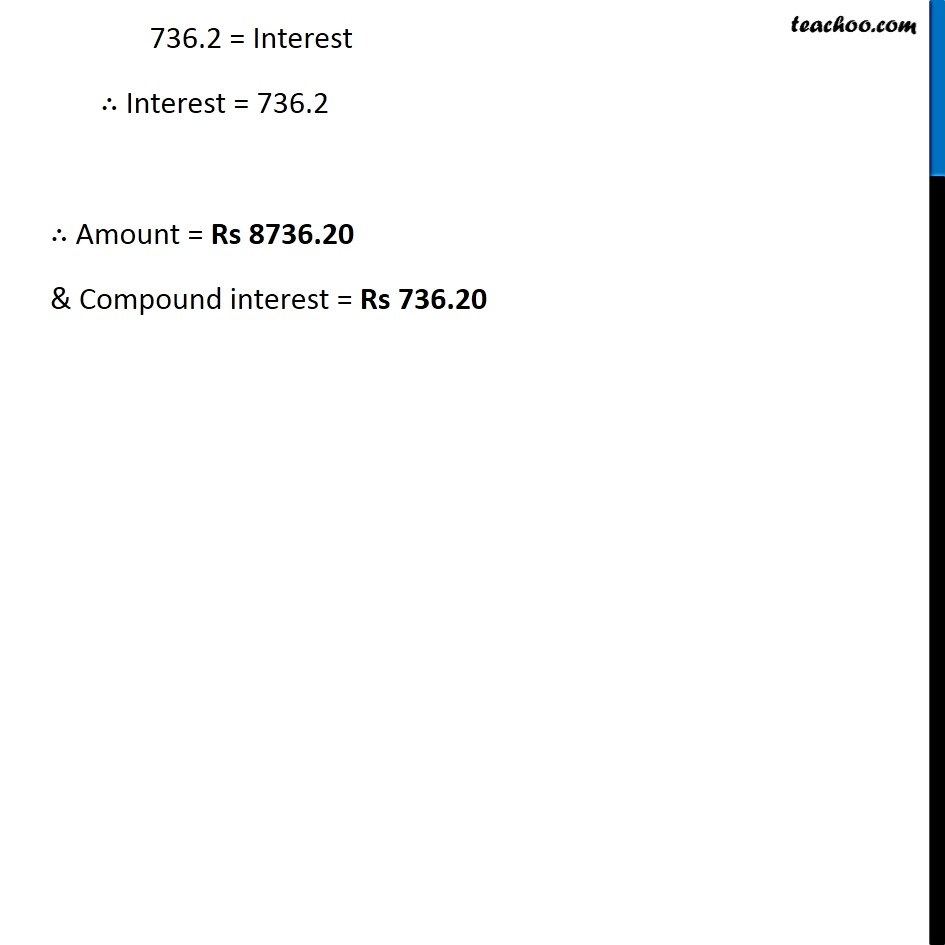Ex 7.3

Chapter 7 Class 8 Comparing Quantities
Serial order wiseLearn in your speed, with individual attention - Teachoo Maths 1-on-1 Class

### Transcript

Question 1 1 (d) Calculate the amount and compound interest on (d) Rs 8,000 for 1 year at 9% per annum compounded half yearly. (You could use the year by year calculation using SI formula to verify). Given, Principal (P) = 8,000 Rate (R) = 9% per Annum Compounded half Yearly = 9/2 % Per half year. Time (N) = 1 Year = 2 half years. Now, Amount (A) = P (1+𝑅/100)^𝑛 = 8000 (1+(9/2)/100)^2 = 8000 × (1+9/(2 × 100))^2 = 8000 × (1+9/200)^2 = 8000 × ((200 + 9)/200)^2 = 8000 × (209/200)^2 = 8000 × (209 × 209)/(200 × 200) = 8 × (209 × 209)/(2 × 20) = 8 × (209 × 209)/40 = 8/4 × (209 × 209)/10 = 2 × (209 × 209)/10 = (418 × 209)/10 = 87362/10 = 8736.2 ∴ Amount = 8736.2 Now, Amount = Principal + Interest 8736.2 = 8000 + Interest 8736.2 − 8000 = Interest 736.2 = Interest ∴ Interest = 736.2 ∴ Amount = Rs 8736.20 & Compound interest = Rs 736.20